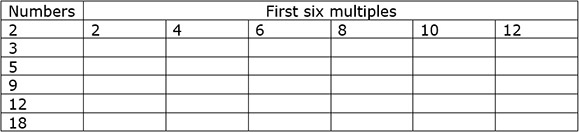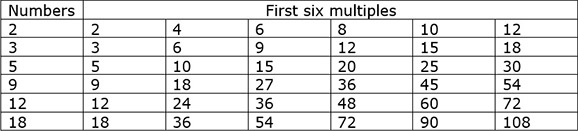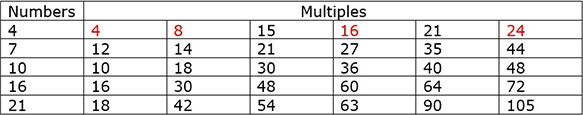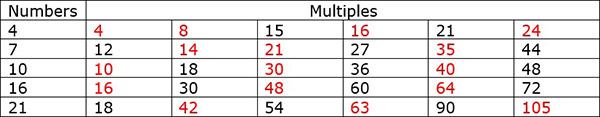# Multiples Problem Type 1

The multiples of a number are numbers obtained by multiplying the number with whole numbers like 1, 2, 3, and so on.

For any given number, there are infinite number of its multiples.

For example, the multiples of 6 are :

6, 12, 18, 24, 30…

Alternatively, multiples can be defined as skip-counting numbers. To find the multiples of five, we skip count by 5s. To find the multiples of ten, we skip count by 10s. To find the multiples of twelve, we skip count by 12s, and so on.

Write the first six multiples for the numbers below.### Solution

Step 1:

The first six multiples of given numbers are found by multiplying them with whole numbers 1 to 6 or by skip counting by given numbers.

Step 2:

The first six multiples of given numbers are as follows:Select the multiples of the given numbers from the numbers given alongside.### Solution